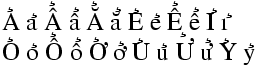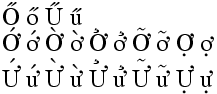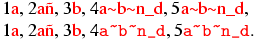# All commands, alphabetic order; letter H

## \h

The \h command puts a hook accent on some letters. These are the possibilities:


\h A \h a \h{\^A} \h{\^a} \h{\u A} \h{\u a} \h E \h e  \h{\^E} \h{\^e}  \h I
\h i \h O \h o  \h{\^O} \h{\^o}  \h{\H O} \h{\H o}  \h U \h u
\h{\H U} \h{\H u} \h Y \h y


The XML result is

&#x1EA2; &#x1EA3; &#x1EA8; &#x1EA9; &#x1EB2; &#x1EB3; &#x1EBA; &#x1EBB; &#x1EC2;
&#x1EC3; &#x1EC8; &#x1EC9; &#x1ECE; &#x1ECF; &#x1ED4; &#x1ED5; &#x1EDE; &#x1EDF;
&#x1EE6; &#x1EE7; &#x1EEC; &#x1EED; &#x1EF6; &#x1EF7;


Preview (not wonderful)The HTML characters Ả ả Ẩ ẩ Ẳ ẳ Ẻ ẻ Ể ể Ỉ ỉ Ỏ ỏ Ổ ổ Ở ở Ủ ủ Ử ử Ỷ ỷ

## \H

The \H command puts a Hungarian accent on some letters. It puts also a horn on some letters (used with other accents). These are the possibilities:


\H O \H o \H U \H u\\
\H{\'O} \H{\'o}  \H{\O} \H{\o}  \H{\h O} \H{\h o}  \H{\~O} \H{\~o}
\H{\d O} \H{\d o}\\ \H{\'U} \H{\'u} \H{\U} \H{\u} \H{\h U} \H{\h u} \H{\~U}
\H{\~u} \H{\d U} \H{\d u}


The XML result is

&#336; &#337; &#368; &#369;
&#7898; &#7899; &#7900; &#7901; &#7902; &#7903; &#7904; &#7905;
&#7906; &#7907;
&#7912; &#7913; &#7914; &#7915; &#7916; &#7917; &#7918;
&#7919; &#7920; &#7921;


PreviewThe HTML characters Ő ő Ű ű Ớ ớ Ờ ờ Ở ở Ỡ ỡ Ợ ợ Ứ ứ Ừ ừ Ử ử Ữ ữ Ự ự

## \halign

The \halign command is the TeX primitive for constructing arrays. It is not yet implemented in Tralics.

## \hangafter (internal integer)

The result of \hangafter=70 is that TeX will change hanging indentation after 70 lines. Tralics does not implement hanging indentation. (See scanint for details of argument scanning).

## \hangindent (internal integer)

The effect of \hangindent=5mm is to use 5mm for hanging indentation (see \hangafter above). No implemented in Tralics. (See scandimen for details of argument scanning).

## \hat

The \hat command puts a hat accent over a kernel. It works only in math mode. See also the \acute command.

If you say \hbadness=25, then TeX will show all hboxes with badness greater than 25. This parameter is ignored by Tralics, since no badness is computed. (See scanint for details of argument scanning).

## \hbar (math symbol)

The \hbar command is valid only in math mode. It generates a miscellaneous symbol: <mi>&#x127;</mi> (Unicode U+127, ħ). See description of the \ldots command.

## \hbox

In Tralics, there is no difference between a vbox and an hbox. Hence \hbox is the same as \vbox (however \everyhbox applies only to an hbox.) See \vbox.

## \hb@xt@

This command expands to \hbox to. Do not modify it.

## \hdots (math symbol)

The \hdots command is valid only in math mode. It generates a miscellaneous symbol: <mi>&ctdot;</mi> (Unicode U+22EF, ⋯)

This command is used when \\ is seen in a section title. You can redefine it at your leisure, the default value is a single space. In a construction like \section{a\\[1cm] b}, the optional argument is read, so that the result contains space. You can use \def \@headercr {\space \ignorespaces} if it worries you.

These parameters contain the height of the header and the distance between the header and the text.

## \heartsuit (math symbol)

The \heartsuit command is valid only in math mode. It generates a miscellaneous symbol: <mo>&heartsuit;</mo> (Unicode U+2665, ♥). See description of the \ldots command.

## \@height

This command expands to height. Do not modify it.

## \heightof

This command is defined by the calc package but not implemented in Tralics.

## \hexnumber@

Reads a number, that should be between 0 and 15, and converts it into a hex digit.

## \hfil

According to Knuth, \hfil is essentially equivalent to \hskip 0pt plus 1 fil. In Tralics, this command leaves vertical mode (may start a paragraph) and inserts a <hfil/> element. This element will be replaced by a space inside a figure environment.

## \hfill

The \hfill command is like \hfil, but inserts a <hfill/> element.

## \hfilneg

The \hfilneg command is like \hfil, but inserts a <hfilneg/> element.

## \hfuzz (rigid length)

You can say \hfuzz=5pt, as a result TeX will complain about overfull hboxes only if they are overfull by more than 5pt. This is set to 0.1pt by LaTeX, and to .5pt by \sloppy. Ignored by Tralics. (See scandimen for details of argument scanning).

## \hideskip (rubber length)

Quantity set by TeX to -1000pt plus 1fill. Used for instance in the last exercise of Chapter 18 of the TeXbook.

## \hl

Command from the soul package, that takes an argument and behaves like \textit. For an example, see \ul.

## \hline

The \hline command is valid only inside a table, either at the very start, or after a \\ command. Its effect is to add an horizontal rule over the current row, more precisely, it adds top-border='true' to the attribute list of the first row (if \hline starts an array) or bottom-border='true' to the previous row (the row ended by the \\ command). See description of the \\ command for an example. See also the page on arrays.

## \hlinee (Tralics command)

The \hlinee command is an extension of \hline, and \cline; it takes four arguments spec, above, below and width. The first first argument is empty or a range (from 3 to 4 in the example below); the other arguments are dimensions; the effect is to add a rule of the given width to the cells specified by the range on the current row (or to the row, if no range is given). The user may indicate that there is some space below or above the rule. The command should be given at the start of the table or after a \\, in which case there will be a top rule on the first row, or a bottom rule otherwise; moreover, there will be an attribute indicating the width, in the form border-top-width='3pt' or border-bottom-width='3pt'. Note the special hack; when looking for a rule, Tralics expands the next command; if it has the form \@ifnextchar wAB, the command is evaluated, replaced by one of A and B, and the result is checked again.

\begin{tabular}{llll}
\hlinee{}{}{}{5pt} a&b&c&d\\\hlinee{}{}{}{6pt} \hlinee{3-4}{}{}{7pt}
\end{tabular}

\begin{tabular}{llll}
\hlinee{}{1pt}{2pt}{5pt} a&b&c&d\\\hlinee{}{3pt}{4pt}{6pt} \hlinee{3-4}{-1pt}{-2pt}{7pt}
\end{tabular}


<table rend='inline'><row border-bottom-width='6.0pt' bottom-border='true' border-top-width='5.0pt' top-border='true'>
<cell halign='left'>a</cell>
<cell halign='left'>b</cell>
<cell border-bottom-width='7.0pt' bottom-border='true' halign='left'>c</cell>
<cell border-bottom-width='7.0pt' bottom-border='true' halign='left'>d</cell>
</row></table>

<table rend='inline'><row bottom_rule_space_below='4.0pt'
bottom_rule_space_above='3.0pt' border-bottom-width='6.0pt'
bottom-border='true' top_rule_space_below='2.0pt' top_rule_space_above='1.0pt'
border-top-width='5.0pt' top-border='true'>
<cell halign='left'>a</cell>
<cell halign='left'>b</cell>
<cell bottom_rule_space_below='-2.0pt' bottom_rule_space_above='-1.0pt'
border-bottom-width='7.0pt' bottom-border='true' halign='left'>c</cell>
<cell bottom_rule_space_below='-2.0pt' bottom_rule_space_above='-1.0pt'
border-bottom-width='7.0pt' bottom-border='true' halign='left'>d</cell>
</row></table>



## \hoffset (rigid length)

You can say \hoffset=.5in, as a result TeX will shift by .5in horizontally the box put by \shipout in the dvi file. Not used by Tralics. (See scandimen for details of argument scanning).

## \holdinginserts (internal integer)

When you say \holdinginserts=88 (or any positive number) then insertions remain in place after a page break, otherwise TeX uses the algorithm described at the end of the Chapter 15 of the TeXbook. Unimplemented in Tralics (insertions are very complex objects). (See scanint for details of argument scanning).

## \hom (math symbol)

The \hom command is valid only in math mode. Its translation is a math operator of the same name: <mo form='prefix'>hom</mo>. For an example see the \log command.

## \hookleftarrow (math symbol)

The \hookleftarrow command is valid only in math mode. It generates an arrow pointing to the left with a hook: <mo>&hookleftarrow;</mo> (Unicode U+21A9, ↩). See description of the \leftarrow command.

## \hookrightarrow (math symbol)

The \hookrightarrow command is valid only in math mode. It generates an arrow pointing to the right with a hook: <mo>&hookrightarrow;</mo> (Unicode U+21AA, ↪). See description of the \leftarrow command.

## \hphantom

The three commands \phantom, \hphantom and \vphantom, take an argument, say X, and produce a phantom, i.e., an invisible object that has the same dimension as X. In the case of \hphantom, the height and depth are set to zero, and the command behaves like horizontal space. In the case of \vhphantom, the width is set to zero; such a construct can be used to increase the space between rows in a table. Example:

$\phantom{x} \hphantom{x} \vphantom{x}$


is

<formula type='inline'>
<math xmlns='http://www.w3.org/1998/Math/MathML'>
<mrow>
<mphantom><mi>x</mi></mphantom>
</mrow>
[/itex]
</formula>


## \href, \Href

The \href command takes two arguments, an URL and a text to be typeset; the semantics changed in version 2.2, and \Href was introduced: the effect is the same but the order of arguments changed. Example:

\Href{a}{b} \Href{a\~n}{b} \Href{b}{a\~n}
%\href{b}{a} \href{b}{a\~n} \href{a\~n}{b} alternate syntax


this is translated into

<xref url='b'>a</xref> <xref url='b'>añ</xref> <xref url='a~n'>b</xref>


As this example shows, tilde, underscore and \~ are handled in a special way. It is possible to change the font used for the url. Traditionally, people use the \url command; its effect is to typeset the first argument using the same rules as the second argument, using a tt font. In fact, Tralics puts the value of \urlfont before the argument of \url. This command does nothing, but the user can change its value. Example

{
1\Href{a}{b}, 2\Href{a\~n}{b}, 3\Href{b}{a\~n},
4\Href{\url{a~b\~n_d}}{a~b\~n_d},
5\url{a~b\~n_d},
\let\urlfont\tt
1\Href{a}{b}, 2\Href{a\~n}{b}, 3\Href{b}{a\~n},
4\Href{\url{a~b\~n_d}}{a~b\~n_d},
5\url{a~b\~n_d}.
}


This is the result.

1<xref url='b'>a</xref>, 2<xref url='b'>añ</xref>, 3<xref url='a~n'>b</xref>,
4<xref url='a~b~n_d'>a~b~n_d</xref>,
5<xref url='a~b~n_d'>a~b~n_d</xref>,
1<xref url='b'>a</xref>, 2<xref url='b'>añ</xref>, 3<xref url='a~n'>b</xref>,
4<xref url='a~b~n_d'><hi rend='tt'>a~b~n_d</hi></xref>,
5<xref url='a~b~n_d'><hi rend='tt'>a~b~n_d</hi></xref>.


Preview. We have inserted a line break, in order to show the difference between the two cases. The red color indicates clickable text (of course, you cannot click on the image).## \hrule

You can say \hrule or \vrule followed by a rule specification, that is, a width, a height, a depth. Each specification can be given more than once. For a \hrule the default height is 0.4pt and the depth is zero. For a \vrule, the default width is 0.4pt. The translation of

\hrule \vrule
\hrule width 1pt depth 2pt height  3pt
\vrule width 1pt depth 2pt height  3pt


is

<rule depth='0.0pt' height='0.4pt'/>
<rule width='0.4pt'/>
<rule width='1.0pt' depth='2.0pt' height='3.0pt'/>
<rule width='1.0pt' depth='2.0pt' height='3.0pt'/>


## \hrulefill

Translation of \hrulefill is <hrulefill/>.

## \hsize (rigid length)

You can say \hsize=5in, as a result TeX will use this quantity as the line width. Ignored by Tralics. In LaTeX, the document class should specify \textwidth, from which \columnwidth is deduced (in two column modes, the column width is a bit less than the half of the text width), and from this the \linewidth is deduced. After that \hsize is set equal to \linewidth. The \linewidth parameter can be modified by environments like quote. In two column modes, a float can occupy two columns so that \linewidth is changed to \textwidth and \hsize is changed accordingly. A LaTeX user should neither access nor modify the \hsize variable. (See scandimen for details of argument scanning).

## \hskip, \hglue

The \hskip command scans some glue, for instance \hskip-5cm or \hskip0pt plus 2fil. (for the syntax, see scanglue). In the current version, one &nbsp; (Unicode U+A0) is printed every 4 pt (the glue is first transformed into a dimension, -5cm and 0pt in the example), and negative values are ignored. Thus both examples produce nothing. However, a new paragraph is started in any case. In LaTeX, \hglue is a bit more elaborate.

## \hslash (math symbol)

The \hslash command is valid only in math mode. It generates a miscellaneous symbol: <mi>&hbar;</mi> (Unicode U+210F, ℏ)

## \hspace

The translation of \hspace{xx} or \hspace*{xx} is the same as \hskip xx\relax. See above.

In math mode, \hspace{50pt} translates to <mspace width='50.0pt'/>.

## \hss

The \hss command is like \hfil, but inserts a <hss/> element.

## \ht

A command like \ht0=2\ht1 says to replace the height of the box number 0 by twice the height of box number one. See scanint for how integers are read (here the integer is zero, it should be a number between 0 and 255 or 1023). See scandimen for how dimensions are read (here 2\ht1 is the dimension).

Tralics does not use nor remember box dimensions. In the example above 2\ht1 evaluates to 0pt, and modifying \ht0 does not modify the box.

## \bodytext, \externallabels, \externalref, \internal, \segment, \startdocument, \strikeout, \tableofchildlinks (from html pk)

There are a number of commands defined by the html package that do nothing in LaTeX, and has no effect in Tralics either; they are the following \html{a}, \HTML[x]{a}, \htmladdtonavigation{a}, \htmladdtostyle[x]{a}{b}, \htmladdTOClink[x]{a}{c}{c}, \htmlbase{a}, \htmlbody[x], \htmlborder[x]{a}, \htmlclear[x], \HTMLcode[x]{a}, \htmlhead[x]{a}{b}, \htmlimage[x]{a}, \htmlinfo*[x] \htmlnohead, \htmllanguagestyle[x]{a}, \htmlrule*[foo] \HTMLset[x]{a}{b}, \HTMLsetenv[x]{a}{b}, \htmlsetstyle[x]{a}{b}, \htmltracenv{a}, \htmltracing{a}, These commands behave the same, although their name does not start with html. \bodytext{a}, \externallabels[x]{a}{b}, \externalref[x]{a}, \internal[x]{a}, \segment*[x] \startdcument \strikeout{a} \tableofchildlinks*[x]

The sequence \htmladdimg[foo]{bar} is translated as \includeghraphics{bar}.

## \htmlcite (from html pk)

The construction \htmlcite{a}{b} is equivalent to a \nocite{b}. There may be optional arguments, like \htmlcite[x]{a}{c}, \htmlcite[x]{a}[y]{c}, that translate to a.

If you say \htmlexcludecomments{foo} \htmlincludecomments{bar}, this defines two environments foo and bar, the content of which is excluded or included in the document. The first instruction is the same as \let\foo\comment, and you should not redefine the comment environment.

## htmlonly (environment from html pk)

This environment behaves like the comment environment, the content is ignored.

## \htmlref (from html pk)

The constructions \htmlref{a}{b} \htmlref[b]{a}{c} \htmlref[b]{a}[d]{c} translate to a.

## \htmlurl (from html pk)

The commmand \htmlurl is the same as \url.

## \huge

The \huge command is a command that selects a very large font. For an example of fonts, see \rm.

## \Huge

The \Huge command is a command that selects a very large font. For an example of fonts, see \rm.

## \hypercite (from html pk)

You can say \hypercite[A]{B}{C}{D}{E}. Optional argument must be empty or int, cite, ext, nocite, no. In the first three cases, the translation is the same as C\cite[D]{E} or C\cite{E} in the case where D is empty. In the last three cases, the syntax is different, D is an optional argument, translation is C\nocite{E}.

## \hyperref (from html pk)

You can say \hyperref[A]{B}{C}{D}{E}. Optional argument must be empty or ref, pageref, page, noref, no, hyper, or html. In the first two cases, the translation is the same as C\ref{D}E, in the next two cases C\pageref{D}E, then C (but there are only 4 arguments), and in the last two cases, the translation is D.

## \hyphenchar

If you say \the\hyphenchar\foo, you will get the hyphen char of the font \foo. This is an integer. If you say \hyphenchar\foo=444, you set this to 444. TeX will hyphenate a word (in a current font) only if the \hyphenchar of that font is an integer between 0 and 255, and is a valid reference to a character in that font. If hyphenation occurs, this character will be inserted. The TeXbook says that \- is the same as \discretionary{XX}{}{}, where XX is \char f where f is the \hyphenchar of the current font, if this is valid, and XX is empty otherwise. In Tralics \- is ignored and the hyphen char of a font is zero by default.

## \hyphenation

You could say \hyphenation{man-u-script man-u-scripts ap-pen-dix} in order to explain how to hyphenate some words. But Tralics does no typesetting, hence no hyphenations. As a result, the command and its argument is ignored.

## \hyphenpenalty

When you say \hyphenpenalty=100, then TeX will use 100 as penalty for a line break that occurs at discretionary break where the pre-break text is not empty (for instance \-). Unused by Tralics. (See scanint for details of argument scanning).back to home page © INRIA 2003-2005, 2006 Last modified $Date: 2015/11/27 17:06:16$